# Samacheer Kalvi 7th Maths Solutions Term 3 Chapter 2 Percentage and Simple Interest Intext Questions

Students can Download Maths Chapter 2 Percentage and Simple Interest Intext Questions and Answers, Notes Pdf, Samacheer Kalvi 7th Maths Book Solutions Guide Pdf helps you to revise the complete Tamilnadu State Board New Syllabus and score more marks in your examinations.

## Tamilnadu Samacheer Kalvi 7th Maths Solutions Term 3 Chapter 2 Percentage and Simple Interest Intext Questions

Exercise 2.1
Try These (Text book Page No. 28)

Question 1.
Find the percentage of children whose scores fall in different categories given in table below.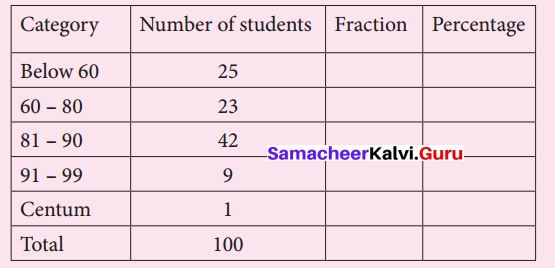Solution: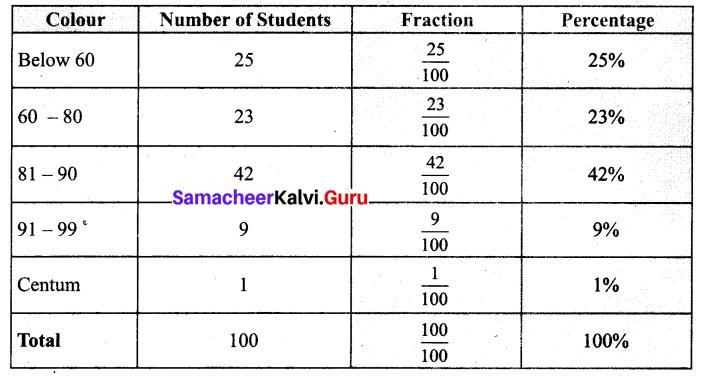Try These (Text book Page No. 29)

Question 1.
There are 50 students in class VII of a school. The number of students involved in these activities are :
Scout: 7
Red Ribbon Club : 6
Junior Red Cross : 9
Green Force : 3
Sports : 14
Cultural activity : 11
Find the percentage of students who involved in various activities.
Solution: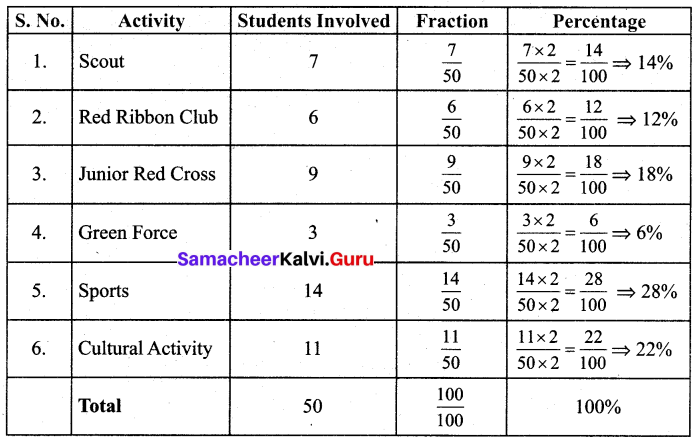Try These (Text book Page No. 30)

Question 1.
Convert the fractions as percentage.
(i) $$\frac { 1 }{ 20 }$$
(ii) $$\frac { 13 }{ 25 }$$
(iii) (i) $$\frac { 45 }{ 50 }$$
(iv) $$\frac { 18 }{ 5 }$$
(v) $$\frac { 27 }{ 10 }$$
(vi) $$\frac { 72 }{ 90 }$$
Solution:
(i) $$\frac { 1 }{ 20 }$$
= $$\frac { 1 }{ 20 }$$ × $$\frac { 100 }{ 100 }$$
= $$\frac { 1 }{ 20 }$$ × 100 %
= 5 %

(ii) $$\frac { 13 }{ 25 }$$
= $$\frac { 13 }{ 25 }$$ × $$\frac { 100 }{ 100 }$$
= $$\frac { 13 }{ 25 }$$ × 100 %
= 52 %

(iii) $$\frac { 45 }{ 50 }$$
= $$\frac { 45 }{ 50 }$$ × $$\frac { 100 }{ 100 }$$
= $$\frac { 45 }{ 50 }$$ × 100 %
= 90 %

(iv) $$\frac { 18 }{ 5 }$$
= $$\frac { 18 }{ 5 }$$ × $$\frac { 100 }{ 100 }$$
= $$\frac { 18 }{ 50 }$$ × 100 %
= 360 %

(iv) $$\frac { 27 }{ 10 }$$
= $$\frac { 27 }{ 10 }$$ × $$\frac { 100 }{ 100 }$$
= $$\frac { 27 }{ 10 }$$ × 100 %
= 270 %

(iv) $$\frac { 27 }{ 10 }$$
= $$\frac { 27 }{ 10 }$$ × $$\frac { 100 }{ 100 }$$
= $$\frac { 27 }{ 10 }$$ × 100 %
= 270 %

(vi) $$\frac { 72 }{ 90 }$$
= $$\frac { 72 }{ 90 }$$ × $$\frac { 100 }{ 100 }$$
= $$\frac { 72 }{ 90 }$$ × 100 %
= 80 %Question 2.
Convert the following percentage as fractions.
(i) 50%
(ii) 75%
(iii) 250%
(iv) 30 $$\frac { 1 }{ 5 }$$ %
(v) $$\frac { 7 }{ 20 }$$ %
(vi) 90 %
Solution:
(i) 50 %
= $$\frac { 50 }{ 100 }$$
= $$\frac { 5 }{ 10 }$$
= $$\frac { 1 }{ 2 }$$

(ii) 75 %
= $$\frac { 75 }{ 100 }$$
= $$\frac { 3 }{ 4 }$$

(iii) 250 %
= $$\frac { 250 }{ 100 }$$
= $$\frac { 25 }{ 10 }$$
= $$\frac { 5 }{ 2 }$$

(iv) 30 $$\frac { 1 }{ 5 }$$ %
= $$\frac{30 \frac{1}{5}}{100}=\frac{\left(\frac{151}{5}\right)}{100}$$
= $$\frac { 151 }{ 500 }$$

(v) $$\frac { 7 }{ 20 }$$ %
= $$\frac{\frac{7}{20}}{100}=\frac{7}{20 \times 100}$$
= $$\frac { 7 }{ 2000 }$$

(vi) 90 % = $$\frac { 90 }{ 100 }$$ = $$\frac { 9 }{ 10 }$$Think (Text book Page No. 32)

Question 1.
What is the difference between 0.01 and 1%.
Solution:
0.01 = $$\frac { 1 }{ 100 }$$ = 1%
0.01 and 1% are the same.

Question 2.
In a readymade shop there will be a board showing upto 50% off. Most of the people will realize that everything is half of its original price, Is that true?
Solution:
No. Only some of them are half of its original price.

Exercise 2.2
Try These (Text book Page No. 33)

Question 1.
Convert these decimals to percentage.
(i) 0.25
(ii) 0.07
(iii) 0.8
(iv) 0.375
(v) 3.75
Solution:
(i) 0.25
= $$\frac { 25 }{ 100 }$$ = 25 %

(ii) 0.07
= $$\frac { 7 }{ 100 }$$ = 7 %

(iii) 0.8
= $$\frac { 80 }{ 100 }$$ = 80 %

(iv) 0.375
= $$\frac { 375 }{ 1000 }$$
= $$\frac { 375 }{ 10 }$$ × $$\frac { 1 }{ 100 }$$
= 37.5 %

(v) 3.75
= $$\frac { 375 }{ 100 }$$ = 375 %Try These (Text book Page No. 34)

Question 1.
Write these percentage as decimals
(i) 3 %
(ii) 25 %
(iii) 80 %
(iv) 67 %
(v) 17.5 %
(vi) 135 %
(vii) 0.5 %
Solution:
(i) 3 %
= $$\frac { 3 }{ 100 }$$ = 0.03

(ii) 25 %
= $$\frac { 25 }{ 100 }$$ = 0.25

(iii) 80 %
= $$\frac { 80 }{ 100 }$$ = 0.8

(iv) 67 %
= $$\frac { 67 }{ 100 }$$ = 0.67

(v) 17.5 %
= $$\frac { 17.5 }{ 100 }$$ = 0.175

(vi) 135 %
= $$\frac { 135 }{ 100 }$$ = 1.35

(vii) 0.5 %
= $$\frac { 0.5 }{ 100 }$$ = 0.005Exercise 2.3
Try These (Text book Page No. 38)

Question 1.
Level of water in a tank is increased from 35 litres to 50 litres in 2 minutes, what is the Percentage of increase?
Solution:
Level of water in the tank originally = 35 litres.
Increase in the water level = amount of change = 50 – 35 = 15 litres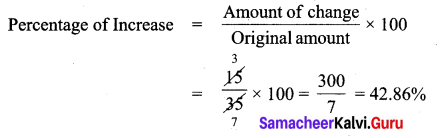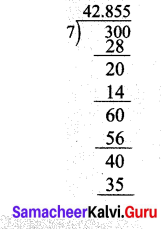Exercise 2.4
Try These (Text book Page No. 41)

Question 1.
Arjun borrowed a sum of ₹ 5,000 from a bank at 5% per annum. Find the interest and amount to be paid at the end of three year.
Solution:
Here Principal (P) = ₹ 5,000
Rate of interest (r) = 5 % Per annum
Time (n) = 3 years
Simple Interest I = $$\frac { pnr }{ 100 }$$
= $$\frac{5000 \times 3 \times 5}{100}$$
= ₹ 750
Amount to be paid A = P + I = ₹ 5,000 + ₹ 750 = ₹ 5,750
I = ₹ 750 ; A = ₹ 5,750

Question 2.
Shanti borrowed ₹ 6,000/- from a Bank for 7 years at 12 % per annum. What amount will clear off her debt?
Solution:
Here principal (P) = ₹ 6,000
Rate of Interest (r) = 12 % Per annum
Time (n) = 4 Years
Simple Interest (I) = $$\frac { pnr }{ 100 }$$ =
= $$\frac{6000 \times 7 \times 12}{100}$$
I = ₹ 5,040
Amount to be paid A = P + I = 6,000 + 5,040 = ₹ 11,040Think (Text book Page No. 43)

Question 1.
In simple interest, a sum of money doubles itself in 10 years. In how many years it will get triple itself.
Solution:
Let the Principal be P and Rate of interest be r % per annum.
Here the number of years n = 10 years
Given in 10 years P becomes 2 P.
A = P + I
After 2 years A = 2P
i.e. 2P = P + I
2P – P = I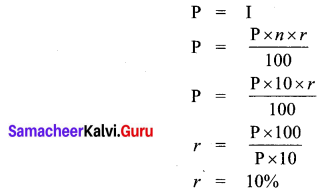Now if the amount becomes triple then A = P + I = 3P
3P = P + I
3P – P = I
2P = I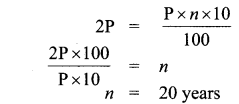∴ After 20 years the amount get tripled.